# 一个非线性系统的混沌现象分析及数值仿真Chaos Analysis and Numerical Simulation of a Nonlinear System

DOI: 10.12677/AAM.2020.93046, PDF, HTML, XML, 下载: 110  浏览: 1,835

Abstract: In this paper, a nonlinear system based on the change of Liu system is discussed, and its chaos is analyzed and simulated. The symmetries, dissipations, singularities and local stability of the system are discussed. The global stability and the existence of attractors are explained. According to the different parameters of the attractor, Poincare section, time series and return map, the chaotic characteristics of the system are described. The simulation results show that the chaotic behavior of the nonlinear system is universal.

1. 引言

1963年，气象学家E.N. Lorenz在数值实验中发现了混沌现象，提出了Lorenz系统 ，自此，不管是国际上还是国内，人们不断地提出和构建新的混沌系统。很多的学术专家都曾展示了自己的研究成果，在国内有影响力的像Chen-系统  ，Lv-系统  等等，在2004年，刘崇新教授等人又提出了Liu-系统 。Liu-混沌系统属于广义Lorenz系统，Chen-系统与Lorenz系统互为对偶系统，而Lv-系统在Lorenz系统和Chen系统之间架起了桥梁，四者之间的联系十分紧密。本文在Liu-系统的基础上，研究了一个新的非线性系统 ，对其动力学行为进行了数值仿真，因而初步分析了其混沌行为   。

2. 数学模型

2.1. 系统描述(1)

2.2. 对称性与不变性

2.3. 系统耗散性与吸引子不变性(2)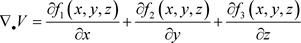(3)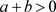时，系统(1)是耗散的，故存在吸引子，即是吸引子存在的条件。

2.4. 平衡点及局部稳定性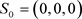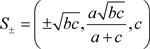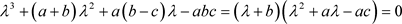(4)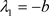,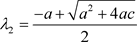,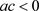时，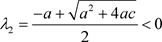，故系统存在三个负实根，此时平衡点稳定。为了直观地判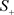对应的Jacobian矩阵为：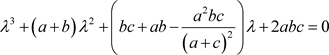(5)

3. 数值仿真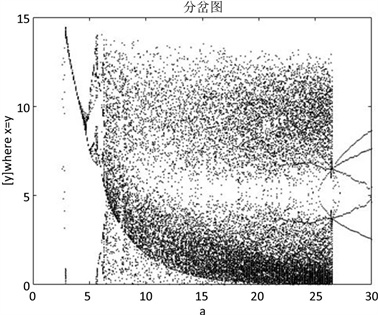Figure 1. Bifurcation diagram when 0 ≤ a ≤ 30, b = 3, c = 13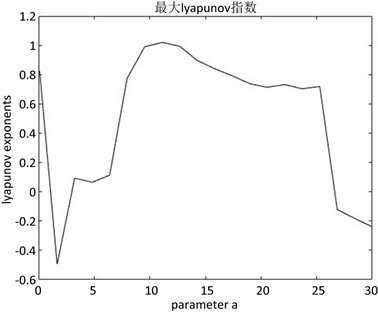Figure 2. Graph of maximum Lyapunov index when 0 ≤ a ≤ 30, b = 3, c = 13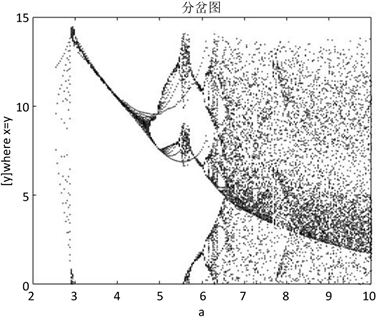Figure 3. Bifurcation diagram when 0 ≤ a ≤ 10, b = 3, c = 13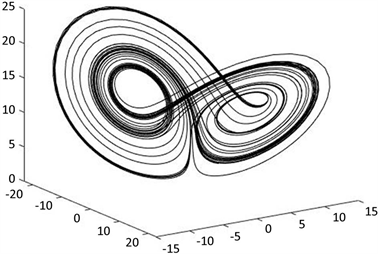Figure 4. Attractor graph when a = 8, b = 3, c = 13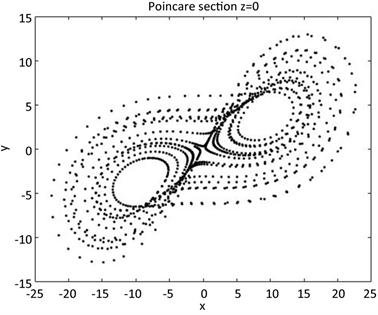Figure 5. Panjialai Section when a = 8, b = 3, c = 13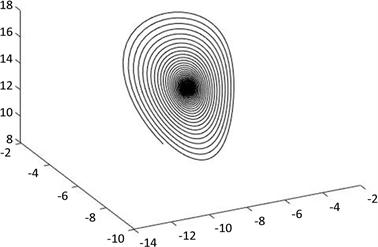Figure 6. Attractor graph when a = 28, b = 3, c = 13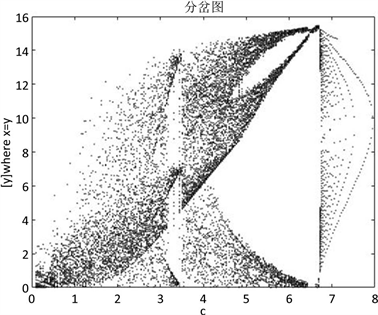Figure 7. Bifurcation diagram when 0 ≤ b ≤ 10, b = 3, c = 13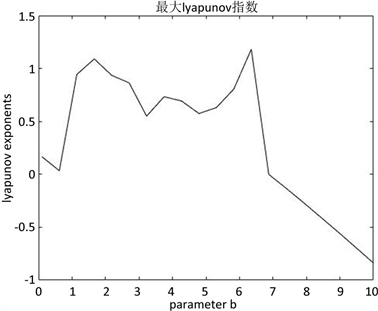Figure 8. Graph of maximum Lyapunov index when 0 ≤ b ≤ 10, b = 3, c = 13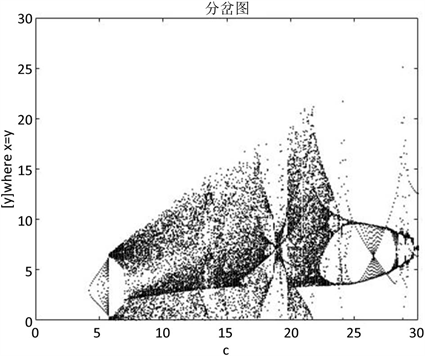Figure 9. Bifurcation diagram when a = 8, b = 3, 0 ≤ c ≤ 30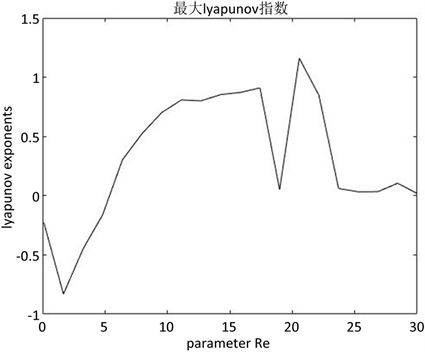Figure 10. Graph of maximum Lyapunov index when a = 8, b = 3, 0 ≤ c ≤ 30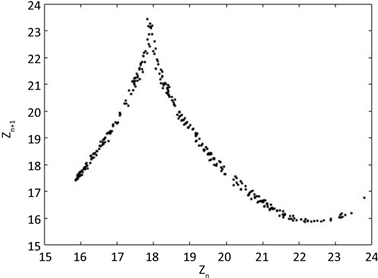Figure 11. Return map when a = 8, b = 3, c = 13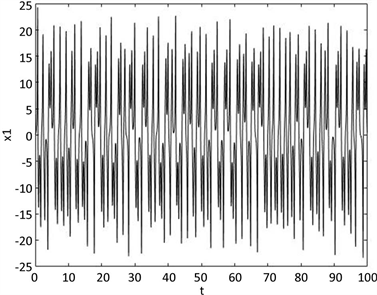Figure 12. Time series when a = 8, b = 3, c = 13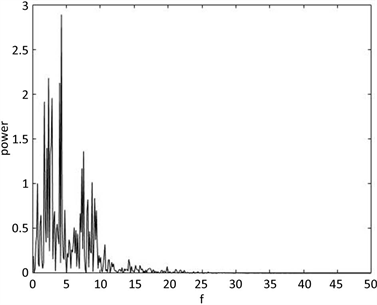Figure 13. Power Spectrum when a = 8, b = 3, c = 13

4. 结论

  Lorenz, E.N. (1963) Deterministic Nonperiodic Flow. Journal of the Atmospheric Sciences, 20, 130-141. https://doi.org/10.1175/1520-0469(1963)020<0130:DNF>2.0.CO;2  Li, T.Y. and Yorke, J.A. (1975) Period Three Implies Chaos. American Mathematical Monthly, 82, 985-992. https://doi.org/10.1080/00029890.1975.11994008  Chen, G, and Ueta, T. (1999) Yet Another Chaotic At-tractor. International Journal of Bifurcation and Chaos, 9, 1465-1466. https://doi.org/10.1142/S0218127499001024  Lv, J.H. and Chen, G.R. (2002) A New Chaotic Attractor Coined. International Journal of Bifurcation and Chaos, 12, 659-661. https://doi.org/10.1142/S0218127402004620  Liu, C.X., Liu, T. and Liu, L. (2004) A New Chaotic Attractor. Chaos, Solitons and Fractals, 22, 1031-1038. https://doi.org/10.1016/j.chaos.2004.02.060  雷腾飞, 付海燕, 陈恒, 窦洋洋. 一类新三维混沌系统的构建及电路仿真[J]. 东莞理学院学报, 2017, 24(1): 23-29.  王贺元. 非线性系统的动力学行为及其数值分析[M]. 北京: 科学出版社, 2018: 114-117.  朱克勤, 彭杰. 高等流体力学[M]. 北京: 科学出版社, 2017: 36-42.  刘秉正, 彭建华. 非线性动力学[M]. 北京: 高等教育出版社, 2003: 120-129.  王贺元. 平面不可压缩磁流体动力学五模类Lorenz方程组的动力学行为及其数值仿真[J]. 数学物理学报, 2017, 37(1): 199-216.  王贺元, 崔进. 旋转流动混沌行为的全局稳定性分析及数值仿真[J]. 数学物理学报, 2017, 37(4): 785-786.